Quantity: 0

Total: 0,00

0

# Geometric transformations – rotation

### Geometric transformations – rotation

This animation demonstrates geometric rotation, a type of geometric transformation both in plane and space.

Mathematics

Keywords

rotation, geometric transformation, reflection on a point, rotation around a line, rotation around a point, geometry, mathematics

Related items

### Scenes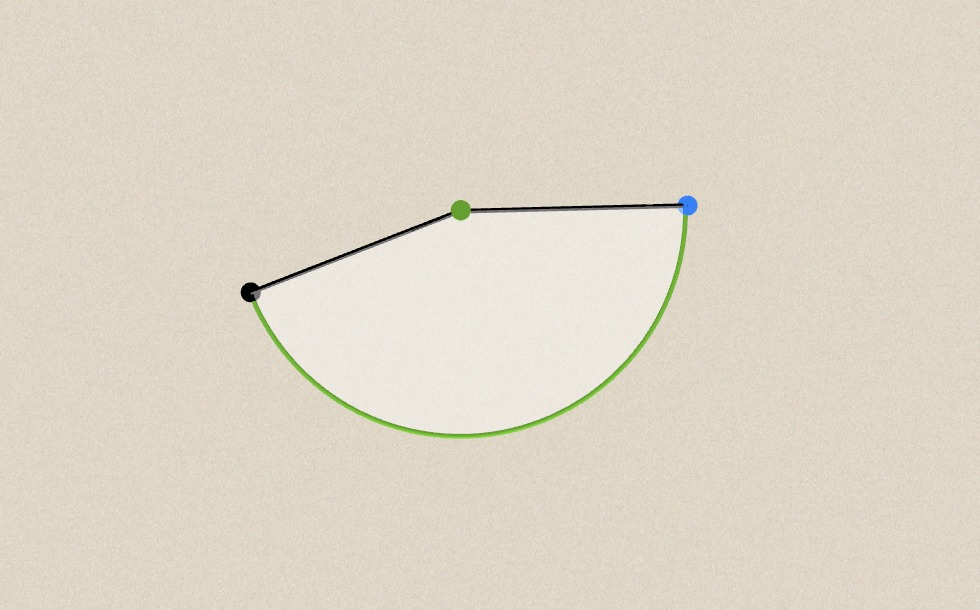### In plane (around a point)

• O
• P
• P'
• α
• x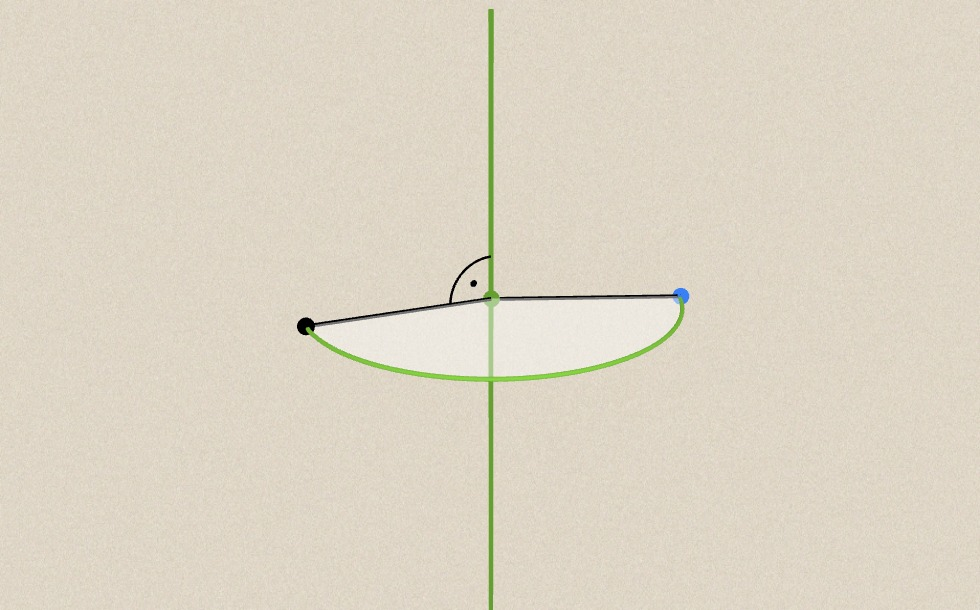### In space (around a line)

• f
• P
• P'
• α
• x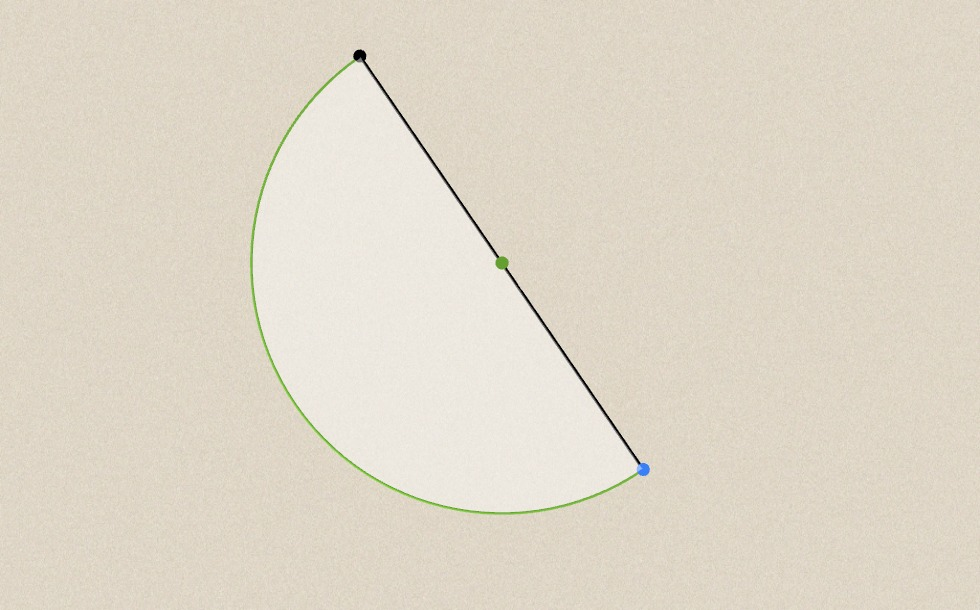### Special case

• P
• P'
• O
• 180°
• point reflection
• x

### Related items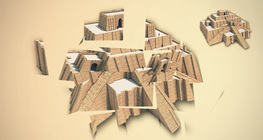#### 3D rotating game

Assemble fragments of broken three-dimensional shapes by rotating the models.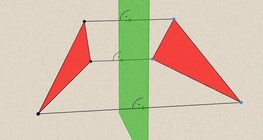#### Geometric transformations – reflection

This animation demonstrates geometric reflection, a type of geometric transformation both in plane and space.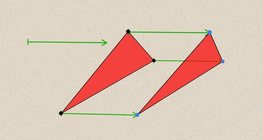#### Geometric transformations – translation

This animation demonstrates geometric translation in both plane and space.#### Solids of revolution

Rotating a geometric shape around a line within its geometric plane as an axis results in a solid of revolution.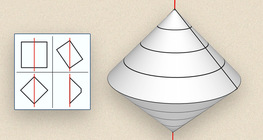#### Solids of revolution (exercise)

An exercise about the generation of solids of revolution.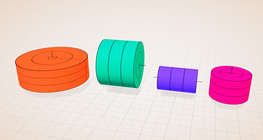#### Solids of revolution (rectangle)

Rotating a rectangle around its axes of symmetry or around its sides results in solids of revolution.#### Angle of inclination of lines and planes

This animation demonstrates the angle of inclination of lines and planes in various positions.#### Relative position of lines and planes

This animation demonstrates the relative position of lines and planes.#### Sphere

A sphere is the set of points which are all within the same distance from a given point in space.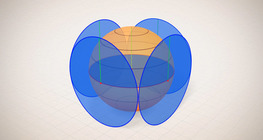#### Surface area of spheres (demonstration)

The surface of a sphere consists of the set of points which are all at the same distance from a given point in space.#### Three-dimensional Cartesian coordinate system

3-dimensional Cartesian coordinate system with illustrations and exercises that develop spatial perception.#### Volume of spheres (Cavalieri´s principle)

Calculating the volume of a sphere is possible using an appropriate cylinder and cone.